# 1. 时间处理

```import time
print time.strftime( '%Y-%m-%d %X', time.localtime())```

python中有四种时间表示方式: 字符串，时间戳，time.struct_time类型，datetime.datetime类型
```#!/usr/bin/env python
# -*- coding: utf-8 -*-
import time
import datetime

def main():
# python中的时间有四种表示方式

# 1.字符串表示
time_string = "20170918123059"
print type(time_string), time_string

# 2.时间戳，一个浮点数，表示从基准时间（1970.01.01 00:00:00）到该时间点所经过的秒数，小数点后面表示毫秒数
time_stamp = time.time()
print type(time_stamp), time_stamp

# 3.time.struct_time类型，该类型其实是一个命名元组(named tuple，类似C语言的结构体)
time_struct = time.localtime()
print type(time_struct), time_struct

# 4. datetime.datetime类型 （还有datetime.date类型，只表示日期，没有时间。）
dt = datetime.datetime.now()
print type(dt), dt

print "================ 互相转换 ==================="

# 时间戳 => struct_time, 使用time.localtime()
st = time.localtime(time_stamp)
print st

# struct_time => 时间戳, 使用time.mktime()
ts = time.mktime(time_struct)
print ts

# struct_time => 字符串, 使用time.strftime(format, t)
str = time.strftime("%Y-%m-%d %H:%M:%S", time_struct)
print str

# 字符串 => struct_time, 使用time.strptime(string, format)
st = time.strptime(time_string, "%Y%m%d%H%M%S")
print st

# datetime => 字符串, 使用datetime.strftime(format)
str = dt.strftime("%Y-%m-%d %H:%M:%S")
print str

# 字符串 => datetime, 使用datetime.strptime(date_string, format)
dt = datetime.datetime.strptime(time_string, "%Y%m%d%H%M%S")
print dt

# datetime => struct_time, 使用datetime.timetuple()
print dt.timetuple()

# datetime => timestamp, 使用datetime.timetuple()得到struct_time类型再转行成timestamp
print time.mktime(dt.timetuple())

# timestamp => datetime, 使用datetime.fromtimestamp()
print datetime.datetime.fromtimestamp(time_stamp)

print "================ 时间差 ==================="

# 计算两个时间的差，最好用时间戳相减，结果即为相差的秒数（小数点后为毫秒）

# 计算上个月的最后一天，可以使用datetime.timedetla类型
now = datetime.datetime.now()
print "当前时间:",  now
print "三小时前:", now - datetime.timedelta(hours=3)
print "三天前:", now + datetime.timedelta(days=-3)
print "上个月最后一天:", datetime.datetime(day=1, month=now.month, year=now.year) + datetime.timedelta(days=-1)

pass

if __name__ == "__main__":
main()
```

# 2. 过程计时

```import timeit
StartTime_timeit = timeit.default_timer()
#procdure
EndTime_timeit = timeit.default_timer()
print "Procedure elapsed ", EndTime_timeit - StartTime_timeit, " seconds"```

# 3. 输出错误信息

```# -*- coding: utf-8 -*-
import sys
import traceback
import logging

def divisionzero():
return 1/0
try:
divisionzero()
except Exception, e:
#打印错误信息
print sys.exc_info()[:2]

#打印traceback信息
print traceback.format_exc()

#打印错误信息
#如果用logger就是logger.error()
logging.error("错误信息: %s", e)

#打印traceback信息
#logging.execption其实是logging.error的升级版，多加了traceback信息
#注意这个方法只能用在except下面
logging.exception("错误信息traceback:")```

# 4. 线程不安全

python有不少模块是线程不安全的。

mysql.connector 的cursor、connector变量也是线程不安全的，千万不要在线程里面共享。

logging模块则是线程安全的。

# 5. 多线程中让主线程等待子线程完成后退出

```def main():

t.start()		#start running a thread in background

# 6. 关于urllib2的坑

(2) 如果在多线程中使用不同代理的话，就不能使用urllib2.urlopen()了，因为urllib2.install_opener() 安装的是一个全局变量。而是应该在线程里直接用opener.open()

```proxy_ip = random.choice(ip_list)
proxy_support = urllib2.ProxyHandler({'http':ip, 'https':ip})
opener = urllib2.build_opener(proxy_support)
req = urllib2.Request(url)
response = opener.open(req, timeout=3)

# 7. 程序入口 if __name__ == '__main__':

python的一个脚本文件，既可以独立执行，又可以当做一个模块被引入另一个文件。

```def test1():
print "i'm in A.test1 function"
return

def test2():
print "i'm in A.test2 function"
return

if __name__ == '__main__':
test1()```

# 8. with ... as ...

```with open("hello.txt") as hello_file:
for line in hello_file:
print line```

```try:
open_file = open('hello.txt')
except:
print 'no such file!'
else:
try:
for line in open_file:
print line
except:
finally:
open_file.close()```

# 9. python的类

## 9.1 class virables与instance virables

class virables有点像类静态变量的意思，所有对象共享该变量（实际上这样讲不严谨，因为对象有可能覆盖一个class virable为instance virable），

instance virables就是普通的变量，每个对象有一份。

## 9.2 私有变量、私有方法、类方法、静态方法

```# -*- coding: utf-8 -*-

class MyClass(object):
# class virables
classVirable = "类变量"

def __init__(self):
super(MyClass, self).__init__()

# instance virables
# 成员变量必须定义在__init__中，如果暂时无值可以用self.variable=None表示
self.variable = "成员变量"
self.__privateVariable = "私有成员变量"
pass

# 双下划线开头为私有
def __privateFunc(self):
print "这个是私有方法"
pass

# python在调用普通实例方法时候，会默认传入实例本身作为第一个实参
# 因此定义类的实例方法，必须定义第一个参数为self
def normalFunc(self):
print "这个是实例方法"
print "可以直接调用私有变量与私有方法:", self.__privateVariable
print self
pass

# python在调用类方法时候，会默认传入类类型作为第一个实参
# 因此定义类的类方法，必须定义第一个参数为clazz
@classmethod
def classFunc(clazz):
print "这个是类方法"
print clazz
print clazz.classVirable
pass

# python在调用类静态方法时候，不会修改要传递的参数
# 因此定义类的静态方法，无需额外增加self或者clazz参数
@staticmethod
def staticFunc():
print "这个是静态方法"
pass

def main():
c = MyClass()

# 类变量，可以通过类名或实例调用，在__init__之前被赋值给实例
# 通过实例修改类变量的话，其实是新建了一个同名成员变量，并不会修改到类变量
print MyClass.classVirable, c.classVirable
MyClass.classVirable = "通过类名修改类变量"
print MyClass.classVirable, c.classVirable
c.classVirable = "通过实例修改类变量"
print MyClass.classVirable, c.classVirable

# 成员变量，不能通过类名调用，只能通过实例调用
print c.variable

# 直接调用私有变量或者私有方法会报错 >> AttributeError: 'MyClass' object has no attribute '__privateVariable'
# print c.__privateVariable
# c.__privateFunc()

# 私有变量与私有方法，在python中其实并不真的私有！而是被改名了
print c._MyClass__privateVariable
c._MyClass__privateFunc()

# 使用实例调用实例方法，python会自动将实例作为第一个参数传入
c.normalFunc()
# 使用类名调用实例方法，必须传递一个实例作为参数
MyClass.normalFunc(c)

# 使用实例调用类方法，python会自动将类类型作为第一个参数传入
c.classFunc()
# 使用类名调用类方法，python会自动将类类型作为第一个参数传入
MyClass.classFunc()

# 使用实例调用静态方法，怎么定义的就怎么调用
c.staticFunc()
# 使用类名调用静态方法
MyClass.staticFunc()
pass

if __name__ == '__main__':
main()```

# 10. 迭代器与生成器(yield)

http://www.ibm.com/developerworks/cn/opensource/os-cn-python-yield/

# 11. 尽量使用logger = logging.getLogger(name)而不是logging

python的logger是有层次关系的，直接使用logging.info()、logging.error()相当于调用的是root logger。logging.getLogger(name)则是从root logger下派生一个名字为name的子logger，一般使用类名进行命名。logging.getLogger()不加参数默认获取root logger。

```import logging

LOGGING_FORMAT = "%(asctime)s - %(levelname)5s - %(name)s: %(message)s"

class AAA(object):
def __init__(self):
super(AAA, self).__init__()
self.logger = logging.getLogger(self.__class__.__name__)

class BBB(object):
def __init__(self):
super(BBB, self).__init__()
self.logger = logging.getLogger(self.__class__.__name__)
self.logger.setLevel("WARNING")

if __name__ == '__main__':
logging.basicConfig(level=logging.DEBUG, format=LOGGING_FORMAT)
logging.info("main func starting")

a = AAA()
b = BBB()

a.logger.info("A INFO")
b.logger.info("B INFO")
b.logger.warn("B WARNING")```

```#!/usr/bin/env python
# -*- coding: utf-8 -*-

import logging
import logging.config

class myHandler(logging.StreamHandler):
"""docstring for myHandler"""
def __init__(self):
super(myHandler, self).__init__()
self.setFormatter(logging.Formatter('%(levelname)5s %(asctime)s %(name)s.%(funcName)s >> %(message)s'))
self.setLevel(logging.WARN)

def main():
logging.basicConfig(level=logging.DEBUG, format='%(asctime)s %(levelname)5s %(name)s.%(funcName)s - %(message)s')
logging.info('main start')

logger1 = logging.getLogger('[logger 1]')
# 实际上是回传给root logger的默认handler来输出
logger1.info('111 test')

logger2 = logging.getLogger('[logger 2]')
# 默认情况下，propagate=True。所有日志都会上传给父logger再处理
# 所以这里如果不关闭propagate，会发现控制台输出了两次日志
logger2.propagate = False

# loghandler的日志等级（如果有设置的话）会自动覆盖logger的日志等级
logger2.setLevel(logging.INFO)

logger2.info('222 info')
logger2.error('222 error')
pass

if __name__ == '__main__':
main()```

# 12. logging.config读取log配置文件

```[loggers]
keys = root

[handlers]
keys = consoleHandler, fileHandler, timedRotatingFileHandler

[formatters]
keys = commonFormatter

############################

[logger_root]
level = NOTSET
handlers = consoleHandler, fileHandler, timedRotatingFileHandler

[handler_consoleHandler]
class = logging.StreamHandler
level = NOTSET
formatter = commonFormatter
args=(sys.stdout,)

[handler_fileHandler]
class = logging.FileHandler
level = NOTSET
formatter = commonFormatter
args=("log/file.log", "a")

[handler_timedRotatingFileHandler]
class = logging.handlers.TimedRotatingFileHandler
level = NOTSET
formatter = commonFormatter
args=("log/rotate.log", 'M', 1)

[formatter_commonFormatter]
format = %(asctime)s %(levelname)5s %(name)s.%(funcName)s - %(message)s
datefmt = %Y-%m-%d %H:%M:%S
# datefmt可以不设置，按asctime的默认格式输出。```

```import logging
import logging.config

class AAA(object):
def __init__(self):
super(AAA, self).__init__()
self.logger = logging.getLogger(self.__class__.__name__)

class BBB(object):
def __init__(self):
super(BBB, self).__init__()
self.logger = logging.getLogger(self.__class__.__name__)
self.logger.setLevel("WARNING")

if __name__ == '__main__':
logging.config.fileConfig("log.config")
logging.info("main func starting")

a = AAA()
b = BBB()

a.logger.info("A INFO")
b.logger.info("B INFO")
b.logger.warn("B WARNING")```

1. FileHandler会继续将日志输出至原有的文件描述符, 从而导致日志切分后日志丢失；
2. WatchedFileHandler会检测文件是否被移动或删除, 如果有, 会新建日志文件, 并输出日志到新建的文件。

# 13. 编码规范

## 13.1 命名规范

package_name, module_name, ClassName, method_name, ExceptionName, function_name, GLOBAL_VAR_NAME, instance_var_name, function_parameter_name, local_var_name.

ps:我以前的代码都是按照c++、java的驼峰方式来命名变量，函数。然后每次PyCharm都有一大堆波浪线提示命名不标准，神烦。=。=#

## 13.2 引号的使用规范

Be consistent with your choice of string quote character within a file. Pick `'` or `"` and stick with it. It is okay to use the other quote character on a string to avoid the need to `\\ `escape within the string.

## 13.3 空格

```Yes: x == 1

No:  x<1```

```Yes: def complex(real, imag=0.0): return magic(r=real, i=imag)

No:  def complex(real, imag = 0.0): return magic(r = real, i = imag)```

# 14. 配置文件解析器ConfigParser

```# 配置文件由多个section组成
# 中括号代表一个section
# section下面跟着它的options
[db]
host = 127.0.0.1
port = 3306
user = root
pass = root

[ssh]
host = 192.168.1.101
user = huey
pass = huey```# 15. 装饰器

python装饰器的语法糖看起来就像java的注解，作用就像面向切面编程。

```# -*- coding: utf-8 -*-
from functools import wraps

def my_decorator(func):
@wraps(func)
def wrapper(*args, **kwds):
print "装饰器 start"
result = func(*args, **kwds)
print "装饰器 end"
return result

return wrapper

@my_decorator
def main():
"""装饰器测试函数"""
print "main process start"
print "main process end"
pass

if __name__ == "__main__":
main()
print "=========================="
# 如果不在上面的装饰器前加@wraps，那么main方法的名字会变成"wrapper"，而且无法找到原本的文档字符串
print "main函数名字：", main.__name__
print "main函数文档字符串：", main.__doc__
```

ps：《用装饰器写一个single instance》

# 16. python的字符编码(the fucking UnicodeEncodeError)

## 16.1 字符集(charset)与字符编码(encoding)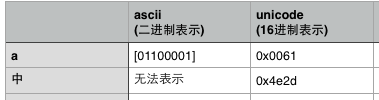ps 1:通常，字符集与字符编码成一一对应关系。比如ascii字符集与字符编码，gb2312字符集与字符编码，但是偏偏unicode字符集却又许多种编码方式，utf-8、utf-16、utf-32都针对unicode字符集的字符编码。

ps 2:字符经过编码后存在内存中的二进制值未必会等值于其在字符集中所规定的二进制值，因为编码可能会对二进制码进行相应的转换。

utf-8编码会对字符的unicode二进制码进行转换后再存储，占用字节数从1~6个字节不等。

utf-16编码同样也会对unicode二进制码进行转换后再存储，占用2或4个字节，同时utf-16编码在存储时候还分大尾序和小尾序。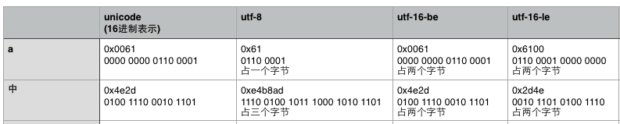## 16.2 <type 'str'>与<type 'unicode'>，decode与encode

python2有两种字符串类型，一种是str字符串(byte string)，一种是unicode字符串(text string)。

(python3的字符串类型不一样了，变成了str类型与bytes类型。str类型即是unicode字符串，bytes类型则表示二进制字符串)

str字符串可以看做是一个字节数组，即将字符串经过字符编码后得到的二进制字节串。（字符编码可以通过encode()方法指定）

unicode字符串则是一unicode字符数组，每个单元就是一个unicode字符。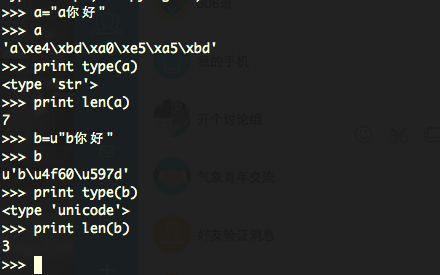python有一对专门的函数来进行byte string与unicode string之间的相互转换：encode()与decode()。

decode()将byte string（str字符串）解码成text string（unicode字符串），二进制码串 >> 解码 >> 字符串
encode()将text string（unicode字符串）编码成byte string（str字符串），字符串 >> 编码 >> 二进制码串

```# -*- coding: utf-8 -*-
print "a你好".decode('utf-8')
print u"a你好".encode('utf-8')

print type("a你好".decode('utf-8'))
print type(u"a你好".encode('utf-8'))

print len("a你好".decode('utf-8'))
print len(u"a你好".encode('utf-8'))```

## 16.3 # -*- coding: utf-8 -*-与sys.setdefaultencoding('utf-8')

```# -*- coding: utf-8 -*-

# coding=utf-8```

# 17. 浮点数转换的坑

int()函数将浮点数转换成整数时，是直接截取整数位，而不考虑小数位是否需要四舍五入的！所以浮点数转换成整数需要先手工四舍五入下再转int。否则遇到99.9999999这种直接int就会变成99而不是100了。

```if __name__ == '__main__':
f = [89.99991, 91.00001, 97.00002, 95.99998]
for i in f:
print i, int(i), round(i), int(round(i))
pass```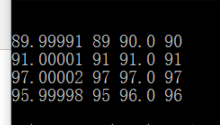# 18. 包、模块与类

## 18.1 导入模块文件

```#!/usr/bin/env python
# -*- coding: utf-8 -*-

import common

def main():
c = common.Common()
c.say_hello()
pass

if __name__ == '__main__':
main()```

```#!/usr/bin/env python
# -*- coding: utf-8 -*-

print "导入本模块的话，这句print会被直接执行"

class Common(object):
"""docstring for Common"""
def __init__(self, arg=None):
super(Common, self).__init__()
self.arg = arg

def say_hello(self):
print "hello, it's common.py Common.say_hello()"
pass
```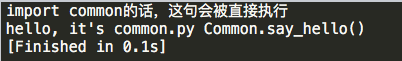## 18.2 import module与from ... import ...的区别

```import common
# 表示导入common模块，
# 这时候想要调用Common类的话，必须加上前缀
# c = common.Common()

from common import Common
# 表示从common模块中导入Common类
# 这时候想要调用Common类的话，只需要
# c = Common()
```

import关键字后面跟的都是module。即使有时候看起来是包名，其实import package等价于import package.__init__，而__init__是这个包的默认模块。

from ... import ...的用法可以是：

from module import Class/func/variable

from package import module

## 18.3 导入包中的模块

`PI = 3.1415926`

```class BasicColor(object):
def __init__(self, rgb=(0,0,0)):
super(BasicColor, self).__init__()
self.rgb = rgb
```

```class BasicShape(object):
def __init__(self, arg):
super(BasicShape, self).__init__()
self.type = arg
```

```#!/usr/bin/env python
# -*- coding: utf-8 -*-

import draw
from draw import colors
from draw.shapes import BasicShape

def main():
print draw.PI

red = colors.BasicColor((1,2,3))
print red.rgb

triangle = BasicShape('triangle')
print triangle.type

pass

if __name__ == '__main__':
main()```

import draw 实际上只是导入了draw包目录下的__init__.py文件。所以可以通过draw.PI来引用draw包的默认模块(__init__.py)中定义的PI变量。但是这句并不会导入除__init__之外的其他模块：colors与shapes模块！！！

from draw import colors 从draw包中导入colors模块。所以可以通过colors.BasicColor来引用colors模块中的类。

from draw.shapes import BasicShape 从shapes模块中直接导入BasicShape类。所以可以直接调用BasicShape类了。

## 18.4 __init__.py的用法

```from .shapes import BasicShape
# 表示从当前包的shapes模块中引入BasicShape类```

## 18.5 绝对路径导入 和 相对路径导入（从兄弟目录导入）

python 支持使用 `from ..sibling_package import foo` 的相对路径方式从兄弟目录中导入模块。但这里其实有个很大的坑！

Note that relative imports are based on the name of the current module. Since the name of the main module is always `"__main__"`, modules intended for use as the main module of a Python application must always use absolute imports.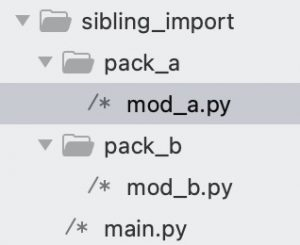```############################################################
# pack_a/mod_a.py
print('A current env: __package__ = %s, __name__ = %s' % (__package__, __name__))
CONSTANT_A = 'AAA'

############################################################
# pack_b/mod_b.py
print('B current env: __package__ = %s, __name__ = %s' % (__package__, __name__))
CONSTANT_B = 'BBB'

############################################################
# main.py
from pack_a.mod_a import *
from pack_b.mod_b import *

if __name__ == '__main__':
print(CONSTANT_A + CONSTANT_B)
print('current env: __package__ = %s, __name__ = %s' % (__package__,__name__))
```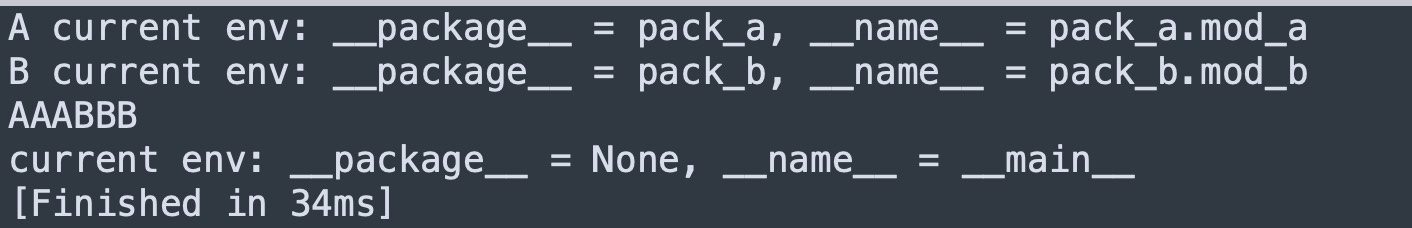（1）如果将 main.py 改为使用相对路径导入。

```from .pack_a.mod_a import *
from .pack_b.mod_b import *

if __name__ == '__main__':
print(CONSTANT_A + CONSTANT_B)
print('current env: __package__ = %s, __name__ = %s' % (__package__,__name__))```

（2）再来，如果我们同时修改 mod_b.py 和 main.py

```############################################################
# pack_b/mod_b.py
print('B current env: __package__ = %s, __name__ = %s' % (__package__, __name__))
CONSTANT_B = 'BBB'

from pack_a.mod_a import *
CONSTANT_BA = CONSTANT_B + CONSTANT_A

if __name__ == '__main__':
print(CONSTANT_BA)

############################################################
# main.py
from pack_a.mod_a import *
from pack_b.mod_b import *

if __name__ == '__main__':
print(CONSTANT_BA)
print('current env: __package__ = %s, __name__ = %s' % (__package__, __name__))```

（3）再来，这时候如果我们把 mod_b.py 导入mod_a 的语句改为相对导入：`from ..pack_a.mod_a import *`

```from sibling_import.pack_a.mod_a import *
from sibling_import.pack_b.mod_b import *

if __name__ == '__main__':
print(CONSTANT_A + CONSTANT_B)
print('current env: __package__ = %s, __name__ = %s' % (__package__, __name__))```

（4）如果非想维持（2）中的 mod_b.py 代码，直接 `from pack_a.mod_a import *` 导入兄弟包中的模块。那么有一个 trick 的方法。就是在导入兄弟包之前，使用 `sys.path.append` 将父目录添加到运行时环境变量中。

```print('B current env: __package__ = %s, __name__ = %s' % (__package__, __name__))
CONSTANT_B = 'BBB'

import sys, os
sys.path.append(os.path.dirname(os.path.dirname(__file__)))
from pack_a.mod_a import *
CONSTANT_BA = CONSTANT_B + CONSTANT_A

if __name__ == '__main__':
print(CONSTANT_BA)
```

（5）但上面的方法（4）实在是有点肮脏 =。=，所以并不推荐在项目的正规代码中使用（我觉得可以在 tests/test.py 中使用）。有网友推荐用 setup.py 来处理兄弟包导入的问题（https://stackoverflow.com/questions/6323860/sibling-package-imports），但我觉得太复杂了。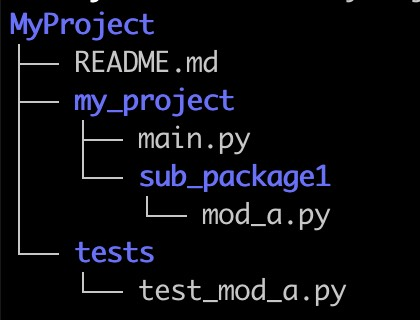① 在项目根目录（或者叫根包，即上图中的 MyProject/my_project/）下，所有子包（MyProject/my_project/sub_package/）内的模块都用 相对导入 的方式来导入兄弟包的模块。（这是针对该项目是独立运行的程序。如果项目是一个要公开的第三方库，似乎更推荐使用 setuptools + pip install -e 的方式，然后直接用 `import my_project.sub_package.module` 的方式来导入。参考 stackoverflow 。）

② 保证不在子包（MyProject/my_project/sub_package/）的模块中编写直接运行的代码 `if __name__ == "__main__":`

③ 所有要直接运行的代码都放在根目录（MyProject/my_project/）下。

④ 对于测试代码。（a）或者放在项目根目录的 Myproject/my_project/tests/ 子目录下；（b）或者放在与项目根目录同级的 MyProject/tests/ 目录中。将项目代码与测试代码分开。

# 19. string.format与数据库操作时候的参数

## 19.1 string.format()用法

```sql_query = "select * from {table_name} where id={id}".format(table_name='user', id=1)
cursor.execute(sql_query)
row = cursor.fetchone()```

## 19.2 cursor.execute()用法

```# 使用%s占位符
cur.execute("""
INSERT INTO some_table (an_int, a_date, a_string)
VALUES (%s, %s, %s);
""",
(10, datetime.date(2005, 11, 18), "O'Reilly"))

# 使用带名字的%(name)s占位符
cur.execute("""
INSERT INTO some_table (an_int, a_date, another_date, a_string)
VALUES (%(int)s, %(date)s, %(date)s, %(str)s);
""",
{'int': 10, 'str': "O'Reilly", 'date': datetime.date(2005, 11, 18)})```

# 20. 动态定义类成员变量

```#!/usr/bin/env python
# -*- coding: utf-8 -*-

class UserModel(object):
fields = ('id', 'name', 'age', 'gender')

def __init__(self, row):
super(UserModel, self).__init__()

assert len(row) == len(self.__class__.fields), '字段数量不一致'

# 通过__dict__动态定义成员变量并赋值
# 等价于定义了 self.id=row, self.name=row, self.age=row
for i in range(len(row)):
field = self.__class__.fields[i]
self.__dict__[field] = row[i]

def main():
user = UserModel((1, 'funway', 18, 'male'))
print(user.name)
pass

if __name__ == '__main__':
main()```

# 21. ftplib的编码问题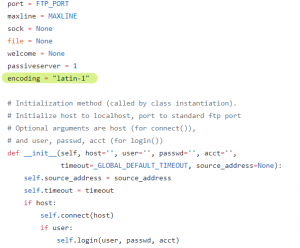1、新建FTP实例后，立即赋值一个encoding成员变量覆盖之（ftplib.py中用到encoding的地方都是用self.encoding来调用，而不是FTP.encoding来调用，所以该方法是有效的）

```def ftp_store():
ftp.encoding = 'utf-8'
file_name = '中文名.txt'
file_data = 'hello,world'
if type(file_data) == str:
file_data = file_data.encode()
ftp.storbinary('STOR %s' % file_name, io.BytesIO(file_data))
pass```

2、每次遇到中文字符串，都主动改成latin-1编码
```def ftp_store():